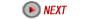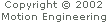### S-Curve Motion Profiles: Command Acceleration vs. Peak Acceleration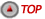The XMP's DSP uses a formula to convert the "commanded acceleration" for an S-Curve move to a "peak acceleration." The DSP calculates the trapezoidal profile first, then adjusts the accel/decel portions of the move based on the "Jerk Percent" parameter, while keeping the move time at a constant value. For example, a 0% jerk would look like a trapezoidal velocity profile (no jerk portion), and a 100% jerk would have no constant accel/decel (all jerks). See the table below. Changes in Jerk Percent parameter change the acceleration-deceleration curve characteristics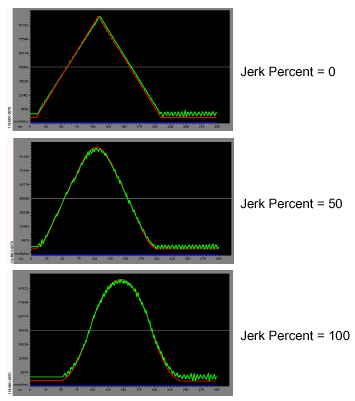The conversion formula is: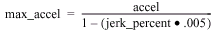where, max_accel is the maximum acceleration (or decel) for a point to point profile accel is the specified acceleration (or decel) from the application code jerk_percent is the specified jerk percentage (0 to 100.0) from the application code.